# Improper Fractions: Lesson for Kids

Instructor: Sabrina Hairston

Sabrina has taught a variety of subjects and grades as a substitute teacher and will complete her MAT in 2016.

In this lesson, you will learn what improper fractions are and how to recognize them. You will also learn how to convert and reduce improper fractions, and we'll review the concept of the greatest common factor.

## What Is an Improper Fraction?

An improper fraction is a fraction whose top number (numerator) is larger than the bottom number (denominator). An improper fraction isn't wrong, but since the numerator, which represents the parts of the whole, is larger, the fraction actually represents a whole number and a fraction.

Examples of improper fractions include: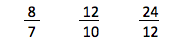## Converting Improper Fractions

To find the whole number in an improper fraction, we can convert, which is to change the fraction to a mixed number. A mixed number has both a whole number and a fraction. For example, if we convert the improper fractions mentioned earlier to mixed numbers, the mixed numbers would look like this: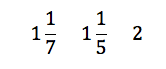How did we do that? In order to convert improper fractions to mixed numbers, there are a couple of steps you must take:

1. First, divide the numerator by the denominator. This will give you a whole number answer.
2. Second, write the remaining value above the denominator. This is the fraction part of your mixed number.

Let's try converting 8/7 into a mixed number. Start by dividing 8 by 7 to get 1. The number 1 then becomes our whole number. The remaining value is also 1. That remaining value is now our new numerator that goes over the denominator (7), giving us the fraction part of the our mixed number.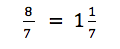## Reducing When Necessary

Sometimes, you'll end up with a mixed number whose fraction is not in the lowest terms, which means the numerator and denominator have no common factor except 1. To bring the fraction to the lowest terms, we reduce the fraction, like in the problem below: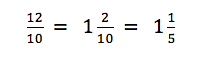Let's work through how we reduce that fraction. We begin by following the same process as before to create our mixed number. We divide 12 by 10 to get a whole number of 1. The remaining value (2) becomes our new numerator, which we place over the unchanged denominator (10). Now, we have our new mixed number: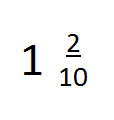To unlock this lesson you must be a Study.com Member.

### Register to view this lesson

Are you a student or a teacher?

#### See for yourself why 30 million people use Study.com

##### Become a Study.com member and start learning now.
Back
What teachers are saying about Study.com

### Earning College Credit

Did you know… We have over 200 college courses that prepare you to earn credit by exam that is accepted by over 1,500 colleges and universities. You can test out of the first two years of college and save thousands off your degree. Anyone can earn credit-by-exam regardless of age or education level.